# Section 1: Understanding the algorithm and designing the GUIDeconvolution

Deconvolution is an integral based mathematical technique that in this case relies on input and output data derived from experimental results:Eq. 1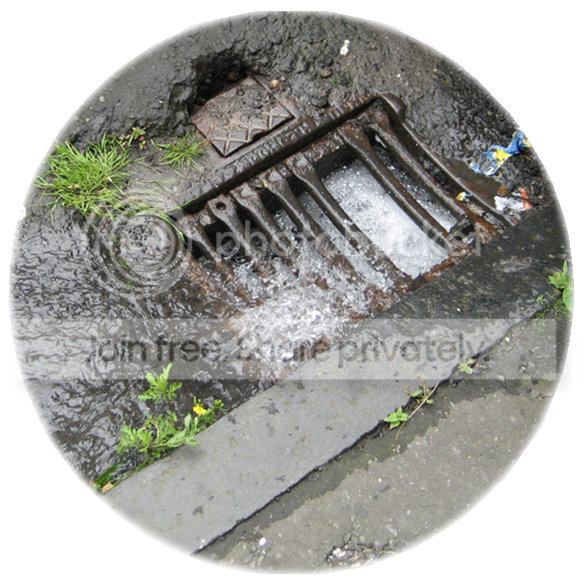Where the variable y(t) is the concentration, u(τ) is the unit impulse response and f (t-τ) can be seen as the input. This will give the output response based on the input.

· This technique works because the function emulates the predictable and natural process of water flow.

· However it is of extreme importance that the input data is accurate with as little ‘noise’ as possible in the data set.

By taking the input data and a predicted set of data determined by the user a function is determined. This function is placed in the convolution integral:

Algorithm theory

· The manhole can be seen as a ‘black box’ which applies a function to an input to give an output.

· The algorithm uses a distribution of contaminant for the input and output, and can calculate the convolution integral required for this process.

· The function of the integral can then be applied to other inputs of the same system to give predicted outputs.Noise

· Noise is fluctuations in and the addition of external factors to the stream of target information being received at a detector.

· The predicted result can be plotted anywhere between these higher and lower boundaries, causing it to vary from the actual result.

· To improve accuracy of results the noise level needs to be predicted so that the algorithm can take this into account. A system with little or no noise will produce a higher accuracy prediction.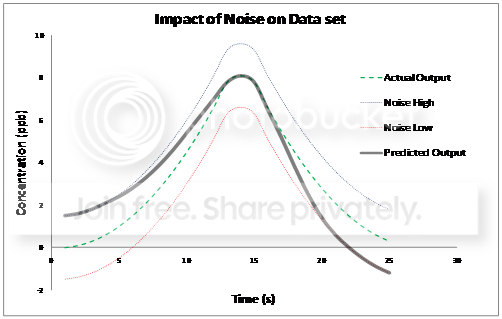Preliminary Plot Placement

· A set of data points are used to model the input distribution, which is used to produce the predicted output.

· To increase speed of iteration as few points are used as possible, therefore an exponential distribution is used.

· This is a limitation to the algorithm as an input of large distribution or occurs later in data set has a lower accuracy estimate.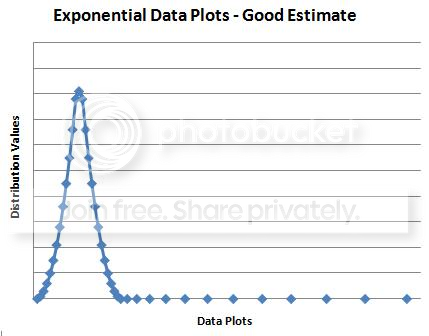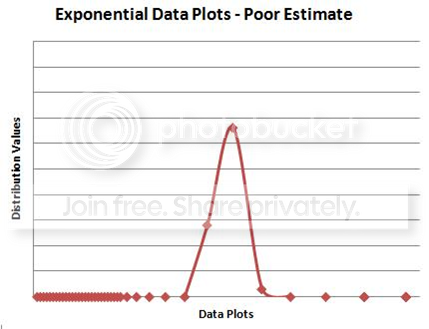Plots show the same data set modelled in different areas of plot distribution, resulting in a good and poor estimate

Creating a Graphical User Interface

For the algorithm to be applied to real world situations by members of industry, or to be used as a teaching tool, it needs to be as simple as possible to operate.

The best way to achieve this is through the creation of a Graphical User Interface or GUI. This allows the user to manipulate parameters and variables within the algorithm without needing to understand the programming language behind it.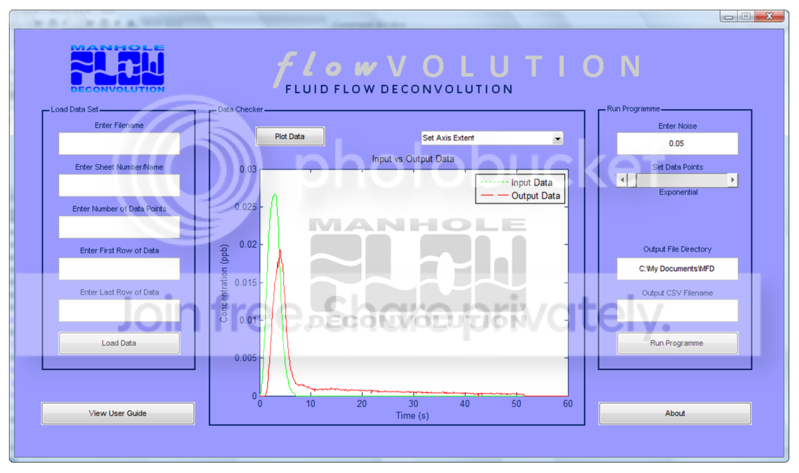The GUI was created in GUIDE, Matlab’s own GUI creator, and is split into three distinct areas:

· The ‘Load Data Set’ area allows the user to specify the exact data for the algorithm to use.

· The ‘Data Checker’ allows the user to plot the loaded data as a graph.

· The ‘Run Programme’ area allows the user to alter parameters within the algorithm.

The GUI will have a built in ‘User Manual’ explaining how the algorithm works.